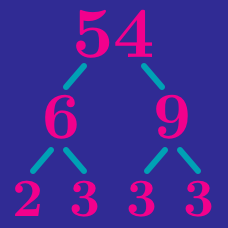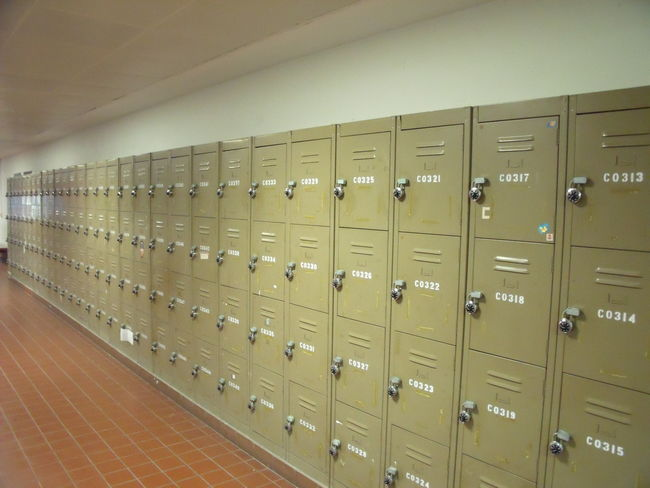Number Theory

Prime Factorization and Divisors: Level 2 Challenges

$\color{#D61F06}{A}$ and $\color{#3D99F6}{B}$ are whole numbers that do not contain 0 as a digit when expressed in base 10

If $\color{#D61F06}{A} \times \color{#3D99F6}{B} = 10000,$ then what is $\color{#D61F06}{A}+\color{#3D99F6}{B}?$A high school has a strange principal. On the first day, he has his students perform an odd opening day ceremony:

There are one thousand lockers and one thousand students in the school. The principal asks the first student to go to every locker and open it. Then he has the second student go to every second locker and close it. The third goes to every third locker and, if it is closed, he opens it, and if it is open, he closes it. The fourth student does this to every fourth locker, and so on. After the process is completed with the thousandth student, how many lockers are open?

Note: The first locker that the $n$-th student changes is the $n$-th locker.

How many trailing zeros are there in the number $3^4 \times 4^5 \times 5^6$?

$\sqrt { { 17 }^{ 2 }+{ 17 }^{ 2 }+{ 17 }^{ 2 }+...+{ 17 }^{ 2 }+{ 17 }^{ 2 }+{ 17 }^{ 2 } }\\ ={ 17 }^{ 2 }+{ 17 }^{ 2 }+{ 17 }^{ 2 }$

How many times should ${17}^{2}$ appear under the square root sign for the equation above to be true?

How many 3 digit numbers have exactly 3 factors?

×